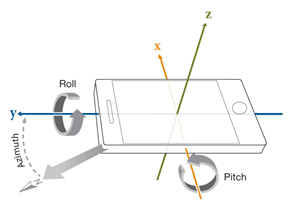# Yaw Pitch & Roll to spherical Theta & Phi

hi guys,
can someone please tell me how to find Theta and Phi from Yaw Pitch and Roll?
I use my smartphone orientation sensor on a project and i need to calculate the smartphone's normal vector projection.

First you must tell us how you want to orient the spherical system in space. Then maybe the question can be answered.

sorry but what do you mean by "orient the spherical system in space"?

Do you want the polar axis to represent your roll axis, or perhaps your yaw axis? Define how the polar coordinate system is to be related to the roll, pitch, and yaw axes.

oh sorry,
yaw is around Z axis
pitch is around x axis
roll is around y axis## 1. What is the difference between Yaw, Pitch, and Roll in relation to spherical Theta and Phi?

Yaw, Pitch, and Roll are terms used to describe the orientation of an object or coordinate system in 3D space. Yaw refers to rotation around the vertical axis, pitch refers to rotation around the horizontal axis, and roll refers to rotation around the forward axis. In contrast, spherical Theta and Phi refer to coordinates on a spherical surface, where Theta represents the angle from the vertical axis and Phi represents the angle from the horizontal axis.

## 2. How can I convert Yaw, Pitch, and Roll values to spherical Theta and Phi values?

There are several mathematical formulas that can be used to convert between Yaw, Pitch, and Roll and spherical Theta and Phi values. One commonly used formula is the Euler angle conversion, where Theta is equal to the inverse cosine of the square root of the sum of the squared sine values of Pitch and Roll, and Phi is equal to the inverse tangent of the ratio of the sine of Pitch to the sine of Theta.

## 3. What is the purpose of converting between Yaw, Pitch, and Roll and spherical Theta and Phi?

Converting between these two coordinate systems can be useful for various applications, such as 3D graphics and navigation systems. It allows for a more intuitive and visually appealing representation of orientation, as well as easier manipulation and calculation of angles.

## 4. Are there any limitations to using Yaw, Pitch, and Roll and spherical Theta and Phi?

While these coordinate systems are commonly used, they do have some limitations. Yaw, Pitch, and Roll can only accurately represent orientation in 3D space if the object does not experience any gimbal lock, which occurs when two of the axes align. Spherical coordinates also have limitations when it comes to representing certain types of motion, such as circular motion, which requires a different coordinate system.

## 5. How can I visualize the conversion between Yaw, Pitch, and Roll and spherical Theta and Phi?

There are various online tools and software programs available that allow you to input values for Yaw, Pitch, and Roll and see the resulting orientation represented in spherical coordinates. Additionally, many 3D graphics programs have the option to toggle between different coordinate systems for visualization.